Olympiad Test Level 2: Numbers’ Magic- 1

# Olympiad Test Level 2: Numbers’ Magic- 1

Test Description

## 10 Questions MCQ Test General Knowledge Olympiad for Class 1 | Olympiad Test Level 2: Numbers’ Magic- 1

Olympiad Test Level 2: Numbers’ Magic- 1 for Class 1 2023 is part of General Knowledge Olympiad for Class 1 preparation. The Olympiad Test Level 2: Numbers’ Magic- 1 questions and answers have been prepared according to the Class 1 exam syllabus.The Olympiad Test Level 2: Numbers’ Magic- 1 MCQs are made for Class 1 2023 Exam. Find important definitions, questions, notes, meanings, examples, exercises, MCQs and online tests for Olympiad Test Level 2: Numbers’ Magic- 1 below.
 1 Crore+ students have signed up on EduRev. Have you?
Olympiad Test Level 2: Numbers’ Magic- 1 - Question 1

### Find the missing number in the given number pattern.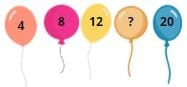Detailed Solution for Olympiad Test Level 2: Numbers’ Magic- 1 - Question 1

4 + 4 = 8
8 + 4 = 12
12 + 4 = 16
16 + 4 = 20

Olympiad Test Level 2: Numbers’ Magic- 1 - Question 2

### Which statement will make this equation complete? 30 ______15 ______ 27

Detailed Solution for Olympiad Test Level 2: Numbers’ Magic- 1 - Question 2

30 > 15 < 27

Olympiad Test Level 2: Numbers’ Magic- 1 - Question 3

### Mukul, Raghav, and Aryan are eating fruits on a hot summer day. Mukul and Raghav do not like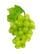grapes. Who likes to eatgrapes?

Detailed Solution for Olympiad Test Level 2: Numbers’ Magic- 1 - Question 3

Aryan likes to eat grapes.

Olympiad Test Level 2: Numbers’ Magic- 1 - Question 4

A rectangle has ______curved line(s).

Detailed Solution for Olympiad Test Level 2: Numbers’ Magic- 1 - Question 4

A rectangle has 0 curved line(s).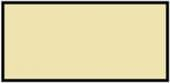Olympiad Test Level 2: Numbers’ Magic- 1 - Question 5

What is the descending order of 67, 87, 77, 57, ?

Detailed Solution for Olympiad Test Level 2: Numbers’ Magic- 1 - Question 5

Descending order is the arrangement of numbers from largest to smallest.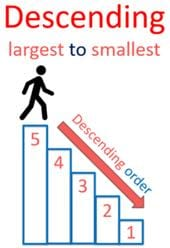Olympiad Test Level 2: Numbers’ Magic- 1 - Question 6

Which of the following is lighter than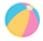?

Detailed Solution for Olympiad Test Level 2: Numbers’ Magic- 1 - Question 6

Eraser is smaller in size than a ball.

Olympiad Test Level 2: Numbers’ Magic- 1 - Question 7

This figure is made up of _______ triangles.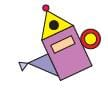Detailed Solution for Olympiad Test Level 2: Numbers’ Magic- 1 - Question 7

This figure is made up of 3 triangles.

Olympiad Test Level 2: Numbers’ Magic- 1 - Question 8

What is the missing number in the given pattern?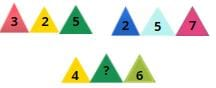Detailed Solution for Olympiad Test Level 2: Numbers’ Magic- 1 - Question 8

3 + 2 = 5
2 + 5 = 7
4 + 2 = 6

Olympiad Test Level 2: Numbers’ Magic- 1 - Question 9

There are _______ groups of 3 pens.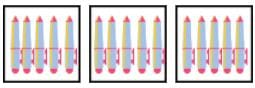Detailed Solution for Olympiad Test Level 2: Numbers’ Magic- 1 - Question 9

Total number of pens is 15.
5 + 5 + 5 = 15

Olympiad Test Level 2: Numbers’ Magic- 1 - Question 10

What comes in between?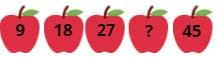Detailed Solution for Olympiad Test Level 2: Numbers’ Magic- 1 - Question 10

9 + 9 = 18
18 + 9 = 27
27 + 9 = 36
36 + 9 = 45

## General Knowledge Olympiad for Class 1

20 docs|39 tests
 Use Code STAYHOME200 and get INR 200 additional OFF Use Coupon Code